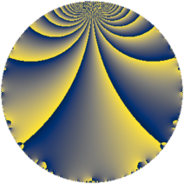Properties

 Label 126.3.rLevel $126$ Weight $3$ Character orbit 126.r Rep. character $\chi_{126}(11,\cdot)$ Character field $\Q(\zeta_{6})$ Dimension $32$ Newform subspaces $1$ Sturm bound $72$ Trace bound $0$

Related objects

Defining parameters

 Level: $$N$$ $$=$$ $$126 = 2 \cdot 3^{2} \cdot 7$$ Weight: $$k$$ $$=$$ $$3$$ Character orbit: $$[\chi]$$ $$=$$ 126.r (of order $$6$$ and degree $$2$$) Character conductor: $$\operatorname{cond}(\chi)$$ $$=$$ $$63$$ Character field: $$\Q(\zeta_{6})$$ Newform subspaces: $$1$$ Sturm bound: $$72$$ Trace bound: $$0$$

Dimensions

The following table gives the dimensions of various subspaces of $$M_{3}(126, [\chi])$$.

Total New Old
Modular forms 104 32 72
Cusp forms 88 32 56
Eisenstein series 16 0 16

Trace form

 $$32 q - 64 q^{4} + 8 q^{6} + 2 q^{7} - 20 q^{9} + O(q^{10})$$ $$32 q - 64 q^{4} + 8 q^{6} + 2 q^{7} - 20 q^{9} - 36 q^{11} + 10 q^{13} + 36 q^{14} + 10 q^{15} + 128 q^{16} - 54 q^{17} + 28 q^{19} + 28 q^{21} - 126 q^{23} - 16 q^{24} + 80 q^{25} - 72 q^{26} - 126 q^{27} - 4 q^{28} + 36 q^{29} + 76 q^{30} + 16 q^{31} - 40 q^{33} - 90 q^{35} + 40 q^{36} + 22 q^{37} + 46 q^{39} + 72 q^{41} + 120 q^{42} + 16 q^{43} + 72 q^{44} + 464 q^{45} - 12 q^{46} + 2 q^{49} - 288 q^{50} - 286 q^{51} - 20 q^{52} - 72 q^{53} - 160 q^{54} - 24 q^{55} - 72 q^{56} - 282 q^{57} - 24 q^{58} - 20 q^{60} + 124 q^{61} + 66 q^{63} - 256 q^{64} - 16 q^{66} - 140 q^{67} + 108 q^{68} + 218 q^{69} + 72 q^{70} + 196 q^{73} + 216 q^{74} + 658 q^{75} - 56 q^{76} + 486 q^{77} + 32 q^{78} + 76 q^{79} - 380 q^{81} - 56 q^{84} + 60 q^{85} - 144 q^{86} - 740 q^{87} - 486 q^{89} + 296 q^{90} - 122 q^{91} + 252 q^{92} + 238 q^{93} - 336 q^{94} + 32 q^{96} - 38 q^{97} + 288 q^{98} + 394 q^{99} + O(q^{100})$$

Decomposition of $$S_{3}^{\mathrm{new}}(126, [\chi])$$ into newform subspaces

Label Dim. $$A$$ Field CM Traces $q$-expansion
$a_{2}$ $a_{3}$ $a_{5}$ $a_{7}$
126.3.r.a $32$ $3.433$ None $$0$$ $$0$$ $$0$$ $$2$$

Decomposition of $$S_{3}^{\mathrm{old}}(126, [\chi])$$ into lower level spaces

$$S_{3}^{\mathrm{old}}(126, [\chi]) \cong$$ $$S_{3}^{\mathrm{new}}(63, [\chi])$$$$^{\oplus 2}$$